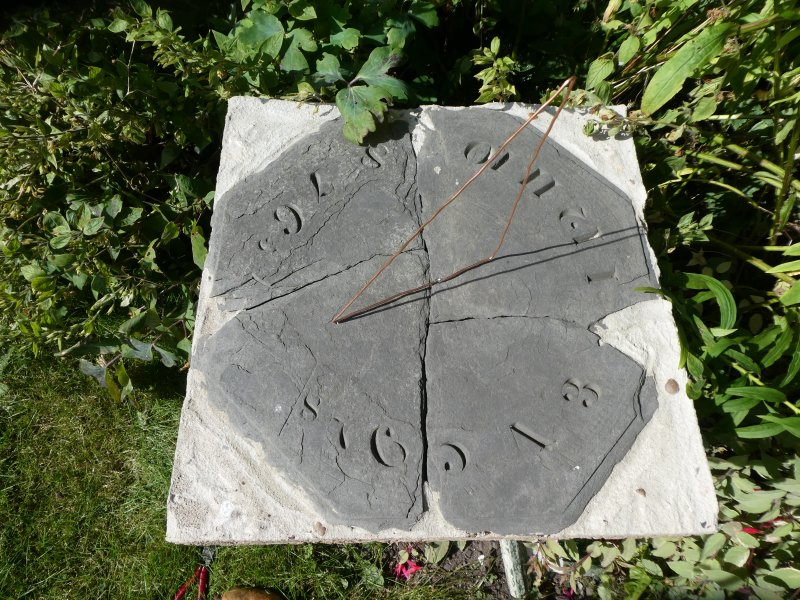When I have had time over the summer, I have got a little more done on the sundial. The Wikipedia page on sundials says that the angle, H,  of the hour lines is given by

tan H = sin θ tan (15° x t)

where θ is the latitude and t is the number of hours before or after noon. I coded up a bit of python to draw the hour lines on a diagram. If I was at the North Pole, then sin θ would be 1 and the lines would be equally-spaced, like this.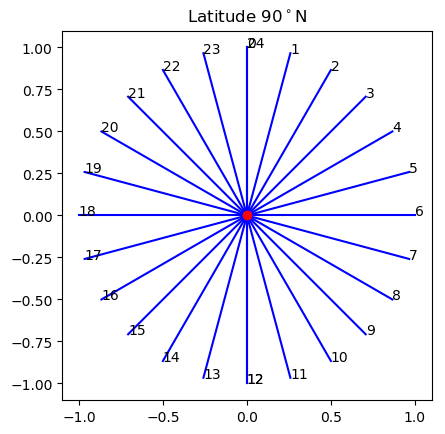If I was exactly at the equator, the gnomon would be horizontal and the lines parallel. If I was near the equator, most of the lines would be bunched together and nearly parallel, like this.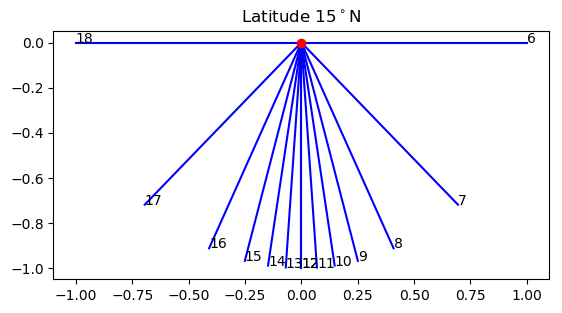Where I live, at 56°N, the lines look like this.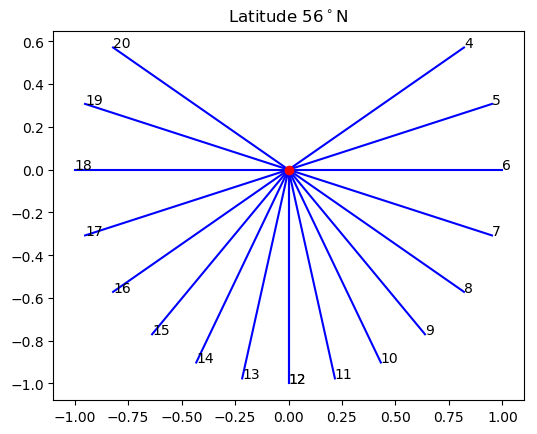In order to locate the point where the gnomon should meet the dial, I printed the hour lines figure on a transparent sheet. I then made it large enough by sellotaping on a second sheet and extending the hour lines with a ruler and felt pen. Here is the result, lined up on top of the sundial.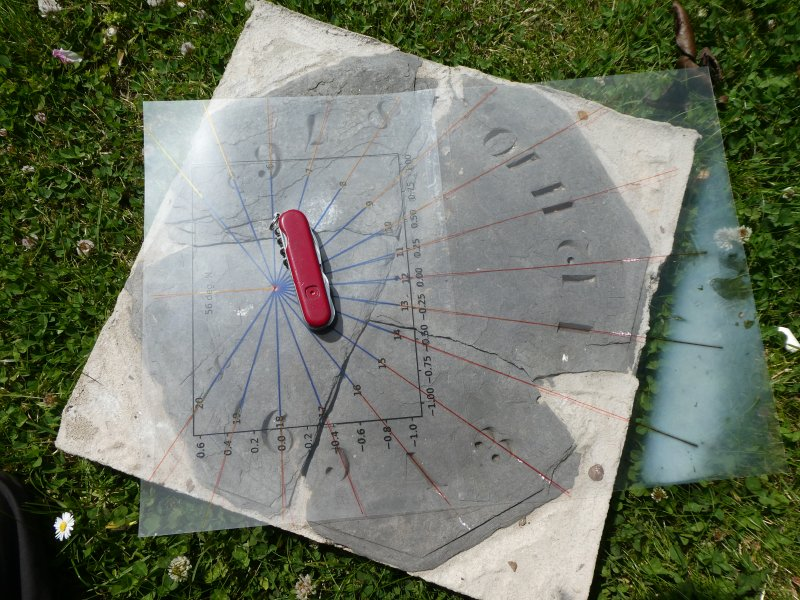With this done, it was easy to stab through the transparency and make a small mark on the dial. I could then construct a gnomon that would meet the dial in the right place. Like the previous attempt it is made of discarded electrical cable and is still rather temporary. Hopefully it will now tell the correct time